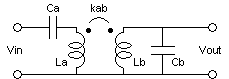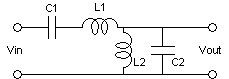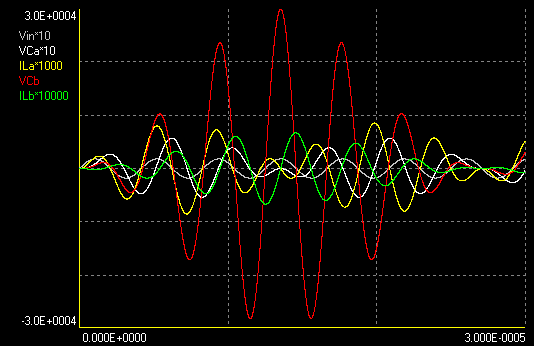## By Antonio Carlos M. de Queiroz

This document complements this other one, discussing possible design procedures for a "double resonance solid-state Tesla coil", but now considering a lossless system . The objective is to design the structure below, when the driving signal is sinusoidal (or a square wave, since this narrow bandwidth system responds essentially only to the fundamental harmonic), requiring that after a certain number of cycles of the input all the energy in the network is concentrated at the output capacitance Cb. This is then equivalent to a Tesla coil, where functionality is achieved through the zero-state response of the network, caused by the input voltage Vin,  instead of by the zero-input response of the classical system, caused by an initial voltage in Ca, with Vin = 0.The design can be more easily done by first designing the transformerless structure below, and then converting it to the final structure through the relations shown. w0 is the "base frequency" in rad/s that multiplies k, l, and m (see below). The usual design would be to specify Ca, Cb, and Lb, and compute w0 and La from the equations. In all cases the coupling coefficient kab depends only on the "mode" k:l:m.w02CbLb = L2C2
w02CaLa = C1(L1+L2)
kab = (L2/(L1+L2))1/2

The circuit is a 4th-order network with two resonance frequencies (pairs of poles at jw in the transfer function), and excitation is at a third frequency. I will assume that these three frequencies are in a ratio k:l:m or three positive integers. I will also consider initially a normalized design where the three frequencies are just k, l, and m rad/s (w0 = 1). Three possible designs that result in complete energy transfer can be identified:

a) The input signal is a cosinusoid, and the three frequencies are in a ratio of successive integers with odd differences (as 1:2:3, or 10:11:14). The excitation is at the central frequency, l. Complete energy transfer occurs in l/2 cycles of the input. The required element values are:

C2 = 1
L2 = 1/l2
L1 = l2/((k2-l2)(l2-m2))
C1 = ((l2-m2)(k2-l2))/(k2m2)

b) The input signal is a sinusoid, and the three frequencies are in a ratio of successive odd integers with double odd differences (as 1:3:5, or 21:23:29). The excitation is at the central frequency, l. This is the most practical design.Complete energy transfer occurs in l/4 cycles of the input. The element values are:

C2 = 1
L2 = (k-l+m)/(klm)
L1 = l(k-l+m)/((k-l)(k+m)2(l-m))
C1 = (l-m)(k+m)2(k-l)/(km(k-l+m)2)

c) The input signal is a sinusoid, and the three frequencies are in a ratio of successive odd integers with double odd differences, as in design (b). The excitation is at the upper frequency, m. This design is usually impractical, but listed here too. Complete energy transfer occurs in l/4 cycles of the input. The element values are:

C2 = 1
L2 = (k-l+m)/(klm)
L1 = -m(k-l+m)/((k-l)2(k+m)(l-m))
C1 = -(l-m)(k+m)(k-l)2/(kl(k-l+m)2)

There is no solution (with positive elements) for excitation at the lower frequency, k.
The voltage gains (maximum Vout / peak Vin) for the three designs can be calculated as:

a)Av = (Ca/Cb)1/2 2km/((k2-l2)(l2-m2))1/2
b)Av = (Ca/Cb)1/2 (km/((l-m)(k-l)))1/2
c)Av = (Ca/Cb)1/2 (kl/((k+m)(l-m)))1/2

When the multipliers k:l:m are successive (increasing by 2 in designs (b) and (c)), these expressions reduce to:

a) Mode k:k+1:k+2: Av = (Ca/Cb)1/2 k(k+2)/(4k2+8k+3)1/2
b) Mode: k:k+2:k+4: Av = (Ca/Cb)1/2 (k(k+4))1/2/2
c) Mode: k:k+2:k+4: Av = (Ca/Cb)1/2 k1/2/2

Designs (a) and (b) generate voltage gains approximately proportional to k. In design (c) the gain increases only with the square root of k.

Note that for these circuits there is always the possibility of driving at one of the resonances, what produces a continuously growing output, that ideally can result in any voltage gain. But the input current grows too at the same rate. For given input and output capacitances, and output inductance, The design procedures (a) and (b) above always reach a given output voltage faster, and with smaller maximum input current. The only problem is that the coupling coefficient may become too small (the voltage gain is inversely proportional to it), and the bandwidth of the system too small too, but the same problem occurs in a system driven at a resonance, if the input must be kept for many cycles. The same idea can be extended for higher-order networks .

Examples:

Sinusoidal input, design (b) mode 11:13:15, Lb=30 mH, Ca=10 nF, Cb=15 pF
Perfect energy transfer in  3.25 cycles.
Normalized transformerless circuit:
C1=   0.0969696970
L1=   0.0625000000
C2=   1.0000000000
L2=   0.0060606061
Final circuit:
Ca=  10.0000000000 nF
La=  49.3636363636 uH
Cb=  15.0000000000 pF
Lb=  30.0000000000 mH
kab=   0.2973176585
Output frequencies: 203172.34, 240112.77, 277053.19 HzThe waveforms above are for 180 V of input peak voltage. The predicted voltage gain is 165.8312395178.
A simulation results in:
Maximum VCa (V) = 571.69140 ( 0.00163 J) at 20.86709 us
Maximum ILa (A) = 8.54905 ( 0.00180 J) at 19.84698 us
Maximum VCb (V) = 29849.64157 (0.00668 J) at 13.53135 us
Maximum ILb (A) = 0.67034 ( 0.00674 J) at 14.54545 us
Maximum voltage gain obtained = 165.83134
Ratio of maximum energies in the capacitors =  4.08927

Note that Ca and La must store only about 1/4 of the output energy. This happens in all practical cases.

It's also possible to design the system with irregular spacing of the three frequencies. If the mode is specified as 23:25:31 (double and add 1 (or -1) to k, l, and m, and then decrease l by 2), complete energy transfer occurs at a "second peak" of the transient waveform envelope, with a somewhat larger voltage gain.

Normalized transformerless circuit:
C1=   0.0583556942
L1=   0.0828760860
C2=   1.0000000000
L2=   0.0065077139
Final circuit:
Ca=  10.0000000000 nF
La=  36.0683367993 uH
Cb=  15.0000000000 pF
Lb=  30.0000000000 mH
kab=   0.2698266359Calculated voltage gain= 199.0254031804
Maximum VCa (V) = 642.53699 (0.00206 J) at 46.46265 us
Maximum ILa (A) = 10.48317 (0.00198 J) at 45.50255 us
Maximum VCb (V) = 35822.55794 (0.00962 J) at 26.12061 us
Maximum ILb (A) = 0.80015 (0.00960 J) at 27.13471 us
Maximum voltage gain obtained = 199.01421
Ratio of maximum energies in the capacitors = 4.66239

If the central frequency is further approximated to one of the border frequencies, the next solution produces a maximum at a "third peak", then at a "fourth peak", and so on. These modes don't seem very practical, however. In the example the output voltage increased by 20%, while the maximum input current increased by 22.6%

A more practical design:

The previous designs didn't produce a high enough output voltage for a spark producing coil. A more powerful system can be obtained by increasing the primary capacitance and using a higher mode. Limiting the coupling coefficient to 0.1, otherwise tuning becomes too critical, the highest mode is 37:39:41 with design b. Complete energy transfer occurs in 9.75 cycles. To obtain 150 kV with 180 V square wave input (a half bridge powered by the rectified 127 V power line, equivalent to 180*4/pi = 229 V), and using the same secondary coil and top load that I used in other practical projects (Lb = 28.2 mH, Cb = 10.4 pF), Ca has to be increased to 12 nF. The final elements are then:
Ca=  12.0000000000 nF
La=  24.6977719183 uH
Cb=  10.4000000000 pF
Lb=  28.2000000000 mH
kab=   0.1021618888
Output frequencies: 279182.274741, 294273.208511 (excitation), 309364.142281 Hz

A simulation results in:
Maximum VCa (V) = -2255.62324 ( 0.03053 J) at  49.25493 us
Maximum ILa (A) =  49.72183 ( 0.03053 J) at 50.13501 us
Maximum VCb (V) = -151526.28034 ( 0.11939 J) at 33.12331 us
Maximum ILb (A) = -2.90453 ( 0.11895 J) at 33.97340 us
Maximum voltage gain obtained = -841.81267
Ratio of maximum energies in the capacitors = 3.91106

In the plot below, the driver is switched off after 10 cycles, and ideal free-wheeling diodes in the half-bridge return most of the energy to the power supply:Note that the input current is in phase with the driving voltage. The use of primary current feedback to ensure zero-current switching on the driver results in the same waveforms, up to the instant when the output voltage is maximum. The driver shall be switched off at this instant, or, with feedback, a cycle leading to three times the input current occurs. Without feedback, the driver returns the energy to the power supply, with the same waveforms shown above. Note that the input current is inverted in the energy return phase.
Excitation at the resonance frequencies results in higher input current for a given output voltage, more cycles to reach the maximum, and input current out of phase with the driving voltage. If primary current feedback is used, the waveforms return to the case of excitation between the resonances shown.

The program drsstcd can make these calculations and simulate the results.
References:
 A. C. M. de Queiroz, Multiple resonance networks with incomplete energy transfer and operating with zero-state response, 2005 IEEE ISCAS, Kobe, Japan, Vol. I, pp. 236-239, May 2005. In IEEE Explore.
 A. C. M. de Queiroz, The triple resonance network with sinusoidal excitation, ICECS 2007, Marrakech, Morocco, pp. 1143-1146, December 2007 (includes some corrections). In IEEE Explore.

Created: 7 September 2004
Last update: 2 June 2020
Developed and Maintained by Antonio Carlos M. de Queiroz